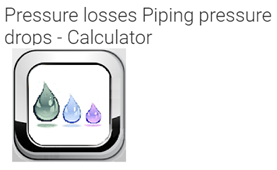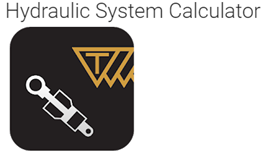# 7 – Best pressure drop calculator apps for android and their features

1. Pressure drop calculatorBy using this app we can calculate the pressure drop of a fluid in a circular pipe due to its friction. In order to check this, we need some input parameters like pipe length, pipe diameter, pipe roughness, flow rate, and physical properties of the flow medium. The pressure drop is calculated by using the Darcy Weisbach equation.

2. Pressure losses piping pressure loss calculatorThis app can be helpful to check the pressure loss and we don’t need an internet connection to use this app, it would work offline. Many piping related calculations can be done by using this app and for each case, this app uses dedicated calculation formulas. We can also share the calculated results by using this app and it will be very useful. This app can do calculations for different fluids, it can calculate the distributed pressure loss for different shapes of pipes like rectangular, circular, etc. it can also do concentrated pressure drop calculation and this app will be useful for piping technicians, designers, hydraulic and aeraulic technicians.This app can calculate the pressure drop of water in a circular pipe and the pressure drop is caused by friction. This app is also used to design a water supply network. Required input parameters are flow, pipe diameter, length of the pipe.

4. Hydraulic system calculatorThis app can be used to calculate the pressure drop around an orifice. This app is capable to calculate the flow rate, pressure, displacement, speed, torque, and power in the motor section. It can also be used to do the calculation of displacement, flow rate, electric motor power, and total efficiency in the pump section.

5. ColebrookThis app can calculate the head-loss in pressure pipe, with the help of Darcy Weisbach formula and Colebrook formula. This app can also be used to calculate the pressure drop.

6. Hydraulic calculationBy using this app we can do many hydraulic calculations of the pipeline. It can do certain functions like calculation of volumetric flow rate of water, calculation of pressure drop on the straight section, it can calculate the total head-loss, etc.